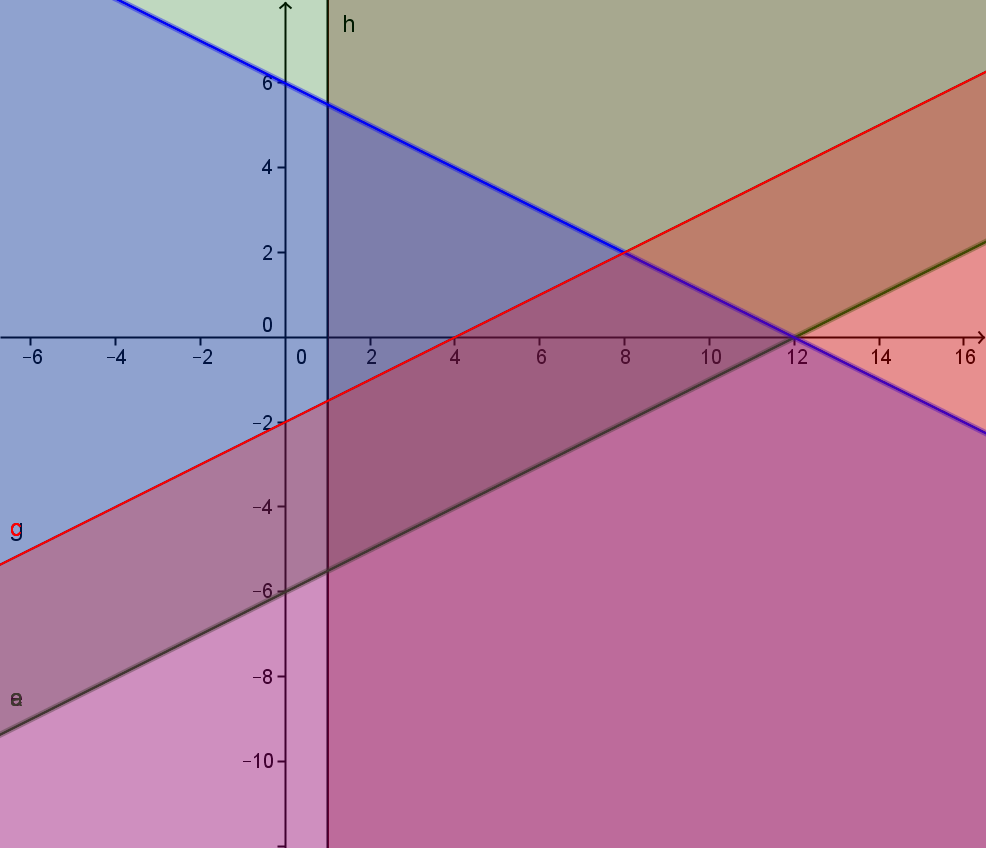## MATH 210 Calculus III Spring, 2015 MTRF 10:00 -10:50 Problem of the Week (sometimes with hints, etc.)

 #1 Predator Prey   Due Feb. 12 #2 Due March 5. Work for a Moving Particle. #3 Homogeneous functions; motion  Due: April 3 #4 Extremes of linear functions on triangular regions. Due  April 17 #5.Independent Factors in Products. Due May 1 6.*** Curvature   Due TBA

1. Due Feb. 12 Estimating Solutions to Parametric Differential Equations: Predator-Prey Model.
Read Section 9.6 of Stewart especially Example 1, parts a and b related to

Equation 1:
dR/dt = kR - aRW      dW/dt = -rW + bRW.

Read pages 589-591 on Euler's method together with materials from Flashman on Euler's method .

Suppose R(0)=100 and W(0)=10 in Equation 1. Estimate R(4) and W(4) using Euler's method with n = 4 with the following choices for the constants a,b,k, and r.
In each case discuss the quality of your estimate and the relation of these to part a and b to Example 1 of Stewart.
1. a = b = 0.001,  k = 0.05,  = 0.01 .
2. a = 0.002,  b =0.05,  k = r = .01.

2.  Due March 5. Work for a Moving Particle.A manually controlled particle moves along the plate in straight lines periodically adjusting the direction vector of the line it follows so that at the time the direction is adjusted the new direction vector is in the direction of vector v= < 2x -y +1, x-2y-1> when at position with coordinates (x,y) and the particle has speed equal to ||v||. The units are feet.  Suppose that during this motion a constant force F= <3,4> is applied to the particle.
i) Describe the motion of the particle from time t= 0 to t= 2 if it is located at (0,0) when t=0 and adjustments are made when t = 0 and t = 1. Draw a figure to illustrate the motion of the particle. Find the work done in moving the particle during the time interval [0,2].
ii) Describe the motion of the particle from time t= 0 to t= 2 if it is located at (0,0) when t=0 and adjustments are made when t = 0,1/2,1, and 3/2,2. Draw a figure to illustrate the motion of the particle.Find the work done in moving the particle during the time interval [0,2].

3.Due Friday April 3. Homogeneous functions and the motion controlled by a heat seeking process.
A. A real valued function of two variables, $f : R^2 \to R$  is called homogeneous of degree $n$ if for any real numbers $x,y$,and $t$,  $f(tx,ty) = t^n f(x,y)$.
For example: $h(x,y) = x^3-3x^2y+5xy^2 -7y^3$ is homogeneous of degree 3.

i.Suppose $f$ is differentiable and homogeneous of degree 2, show that $x D_x f(x,y) + y D_ y f(x,y)= 2 f(x,y)$. [Hint: If $g(t) = f(tx,ty)$ , find $g'(1)$.]

ii. Generalize your result to homogeneous differentiable functions of  degree 3, 4, and $n$.

B. The temperature distribution on a metal plate at time $t$ is given by the function $H_t$ of $x$ and $y$:

$H_t(x,y) = -[(x-cos(\pi t ))^2 + (y-sin(\pi t ))^2 ]$.
A manually controlled particle moves along the plate in straight lines periodically adjusting the direction vector of the line it follows so that at the time the direction is adjusted the new direction vector is in the direction of most rapid temperature increase at that instant and the particle has speed equal to that most rapid rate.
i) Describe the motion of the particle if it is located at $(0,0)$ when $t=0$ and adjustments are made when $t = 0,1,2,3,4,...$ etc.
ii) Describe the motion of the particle if it is located at $(0,0)$ when $t=0$ and adjustments are made when $t = 0, \frac 12,1,\frac 32, 2,...$ etc.

1. Extremes of linear functions on triangular and Polygonal regions. Due Friday April 17   .

2. Suppose $T$ is a triangular region in the plane.
Suppose $L(x,y) = Ax + By$ where $A$ and $B$ are constants that are not both $0$.
Use the LaGrange theory to explain why the maximum (or minimum ) values of  $L$ for the region $T$ will occur at one of the  vertices of the triangle.

State and justify the analogous result for planar regions bounded by quadrilaterals and pentagons.Apply this work to find the maximum and minimum values of $L(x,y) = 3x + 5y$ when $(x,y)$ satisfy all the following (linear) inequalities:

$x - 2y \le 12$;
$12 \ge x + 2 y$;
$4 \le x- 2y$ ;
and $1 \le x$.

3 Bonus Points:  Generalize this problem to one of the following situations:
a.  A tetrahedron in space with $L(x, y, z) = Ax + By + Cz$ with $A,B,$ and $C$ not all $0$.
b.  A planar region bounded by a polygon with $n$ sides.
Justify your statement and illustrate it with an example.

3. Independent Factors in Products. Due May 1
A. Find $\int \int_R (3x^2+1) (4y^3 +2y +1) dA$ where $R = [1,3] × [0,2]$.
B. Suppose $g$ and $h$ are continuous real valued functions of one variable.
Let $F(x,y) = g(x)h(y)$. Explain why $\int \int_R F(x,y) dA = \int_a^b g(x) dx \int_c^d h(y) dy$ where $R = [a,b] × [c,d]$..
C. Use part B to show that  $\int \int_R exp(-x^2 - y^2) dA = [\int_{-m}^m exp(-x^2) dx]^2$   where $R = [-m,m] × [-m,m]$ .

6.(Due : TBA   ) Curvature for graphs of functions in the plane.
Read Curvature on page 855-6 through Example 3.
1. Parametrize the graph of y = f(x) with x = t and y = f(t).

2. Use formula 9 on page 856 to prove that the curvature of the graph of at (x, f(x)) is given by the formula
k (x) = |f '' (x)| / [1 + (f'(x)2] 3/2 .
3. Find where the graph of y = ln(x) has its largest curvature. [Hint: It may be easier to maximize the square of the curvature.]